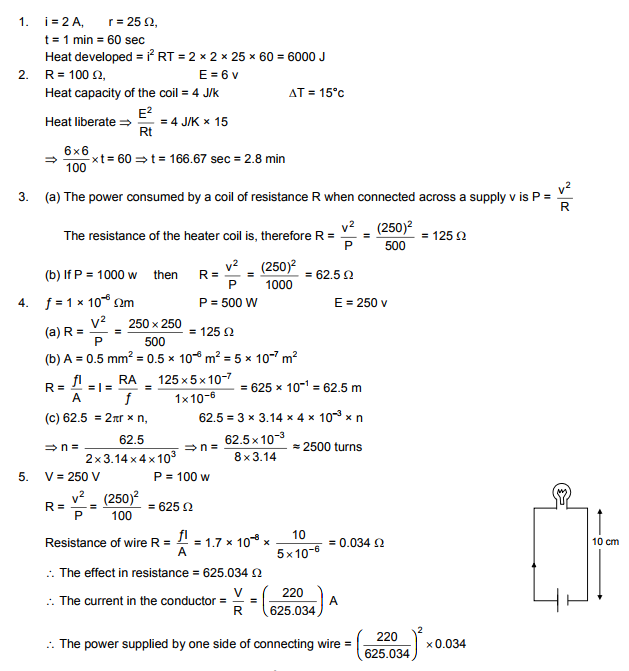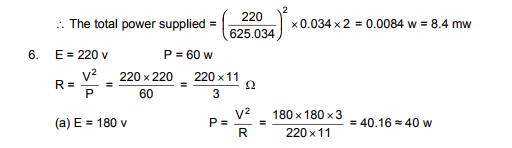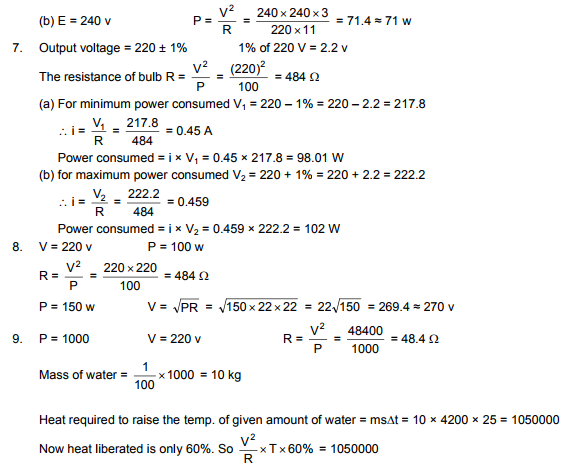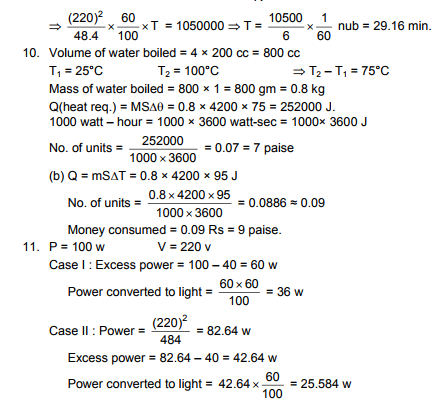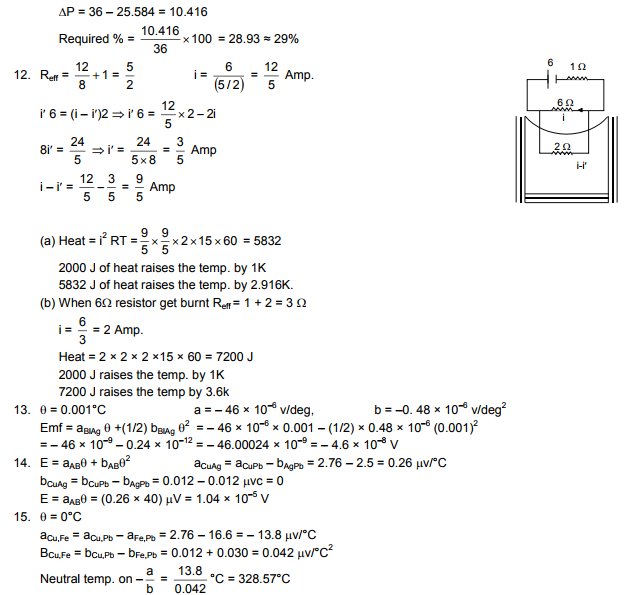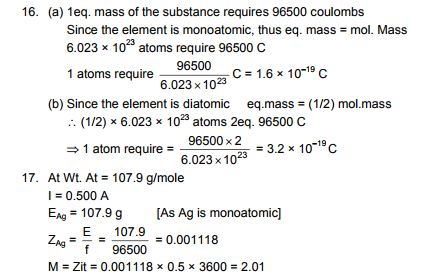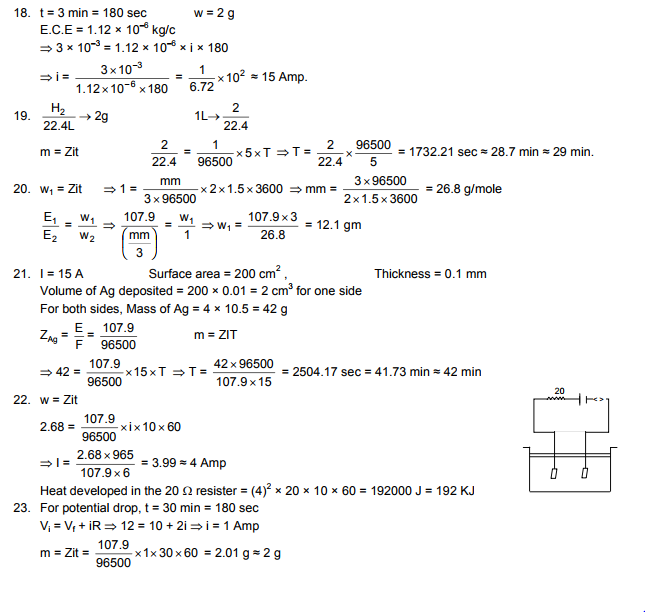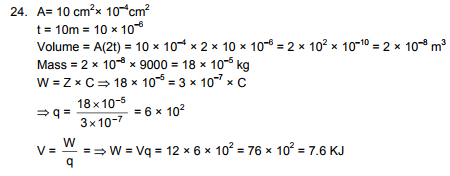# HC Verma Solutions Vol 2 Chapter 11 Thermal And Chemical Effects Of Current

HC Verma Solutions Vol 2 Chapter 11 Thermal and Chemical Effects of Current will help students be more adept and skilled in solving questions that are discussed in the chapter. The solutions have been prepared by our experts and they have provided accurate answers to all the exercises which students can refer and be ready to solve questions at the time of their examinations. This chapter introduces students to a lot of topics related to electric current.

## Main Concepts Related To Thermal And Chemical Effects Of Current

Some of the main topics are;

• Joule’s Law of Heating
• Seebeck Effect
• Peltier Effect
• Thomson Effect
• Electrolysis
• Voltameter and Coulombmeter
• Primary and Secondary Cells

Students will also have to get familiar with mathematical expressions such as I= Q/ t where Q in coulomb and t is in seconds. Complex electric current questions can be solved easily by referring the HC Verma Solutions provided here. Class 12 students can further use this study material for various competitive exams like IIT JEE and NEET.

## Important Questions In Chapter 11

1. We pass a current through a resistor and as a result its temperature increases. Can you define it as an adiabatic process?

2. Will the electrodes in an electrolytic cell have fixed polarity like a battery? Explain with suitable reasons.

3. When heat is developed in a system it is directly proportional to the current flowing through it. State what kind of heat it is.

(a) Thomson heat (b) Peltier heat (c) Joule heat. (d) It cannot be any of these.

4. What does Faraday constant depend on?

(a) The amount of the electrolyte (b) The current in the electrolyte (c) The amount of charge passed through the electrolyte (d) depends on is a universal constant

5. An iron and copper strip are joined at A. The junction A is maintained at 0°C and the free ends X and Y are maintained at 100°C. Where is the potential difference present?

(a)  between the free ends B and C (b) between the two ends of the copper strip (c) between the two ends of the iron strip (d) between the copper end and the iron end at the junction

## HC Verma Solutions Vol 2 Electric Current Chapter 11